# February 15, 2013 Class Notes

## Where we are

• You have some basic modeling terminology:
• Response variable
• Explanatory variables
• You have a way to measure the amount of variation
• Variance in the response variable. You can consider analogous ways, e.g. standard deviation, IQR, 95% range, range, but the variance is standard and for good reasons
• You have a way to measure “how much variation” your model has “explained”
• Variance of the fitted model values
• You have a way to measure how much variation remains unexplained
• Variance of the residuals
• You understand that — when measured with the variance — models partition variation between the fitted model values and the residuals.

## ACTIVITY

Demonstrate the partitioning of variation.

1. Open a document with Rmd.
2. In that document read in some data and fit a model to it using mm
3. Show that the variation is partitioned between fitted and residuals. Show that the variance does this exactly. Then see if the sd or IQR does it.
4. Publish your document to RPubs. Paste the link into Moodle here
5. Now show that adding a new explanatory variable decreases how much variability remains unexplained.
6. In a bit, we're going to do the same with lm

## Models as Functions

We're now going to make a change in perspective. Instead of considering “groups” and assigning the model value based on which group a case belongs to, we're going to think about models as functions.

You are used to using functions when transforming a numerical input to a numerical output, e.g. $$x=A \sin(2\pi t/P)$$ transforms input $$t$$ into an output. So functions are a natural way to include quantitative explanatory variables in a model.

You may not yet be used to treating categorical variables as inputs to a function. That's mainly because the standard algebraic notation (e.g., $$\sqrt(x)$$) doesn't support it. But computer notation can do it in any number of ways.

For instance, we could build a function that takes the group name as an input and returns the corresponding groupwise value.

fetchData("m155development.R")  # load the software

## Retrieving from http://www.mosaic-web.org/go/datasets/m155development.R

##  TRUE

f = listFun(Antelope = 2, Beaver = -1, Cougar = 7)
f("Cougar")

## Cougar
##      7


You won't ever use listFun again, but you will see functions that take categories as inputs.

• There is a standard modeling framework. There are many, many different ways in which you could combine inputs into an output. The one we will use is based in the mathematics of low-order polynomials. For quantitative variables, this is straightforward, no more than $$z = m x + n y + b$$. For categorical variables, imagine that we have a preceeding function that turns each category into a number. Then the following equation would work fine: $z = m x + n y + b + p f(\mbox{animal}).$

• As with polynomials, you can create new “terms” by combining the quantities in various ways. For example, $z = a_0 + a_1 x + a_2 x^2 + a_3 y + a_4 y^2 + a_5 x y + a_6 f(\mbox{animal}) + a_7 x g(\mbox{animal})$ and so on. (Soon, we'll introduce a standard, simple approach to functions of categorical variables, called “indicator functions”.)

• The models are called “linear models” because they are linear combinations of functions of the explanatory variables.

• Some names for these terms:

• intercept — the constant
• main effect — the variable directly, e.g. $$age$$
• interaction term — a product of two (or more) quantities, e.g., $$age \times income$$
• transformation terms — a nonlinear function of a quantity, e.g. $$age^2$$
• Model design: The choice of model terms.

• Model fitting: Finding the best linear combination of the model terms to match the response variable.

## The basic models

Assume we have a response variable D, a quantitative explanatory variable, A, and a categorical variable G. Here are the basic types of models, illustrated on the swimming data.

swim = fetchData("swim100m.csv")

## Retrieving from http://www.mosaic-web.org/go/datasets/swim100m.csv

• D ~ 1

All cases are the same. There is one parameter, the grand mean.

mod = lm(time ~ 1, data = swim)
xyplot(time + fitted(mod) ~ year, data = swim)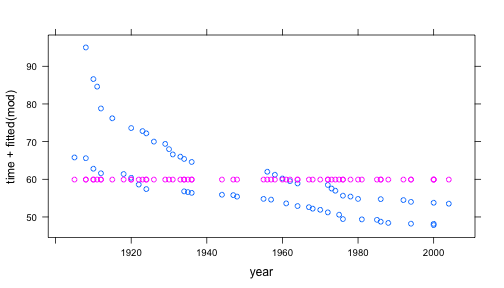• D ~ 1 + A The cases vary with the quantitative variable. There are two parameters: slope and intercept.
mod = lm(time ~ 1 + year, data = swim)
xyplot(time + fitted(mod) ~ year, data = swim)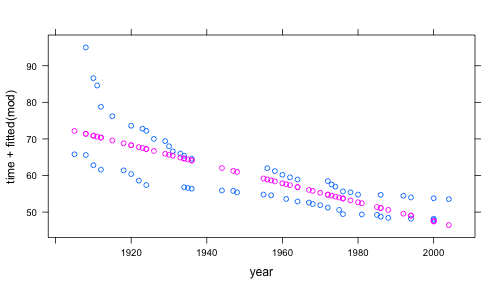• D ~ 1 + G The groupwise mean model. But note that the parameters are written differently, as an “intercept” and a “difference”. QUESTION: Which difference is it?
mod = lm(time ~ 1 + sex, data = swim)
xyplot(time + fitted(mod) ~ year, data = swim)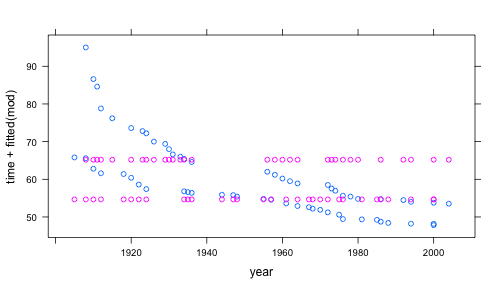• D ~ 1 + A + G Both variables play a role. The parameters are “intercept,” “slope,” and “difference between groups.”
mod = lm(time ~ 1 + year + sex, data = swim)
xyplot(time + fitted(mod) ~ year, data = swim)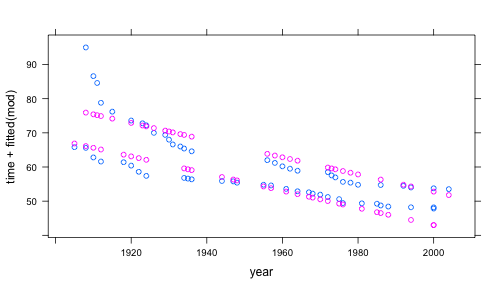Note that the slope is the same for the two groups.

To see a different slope for the two groups, we have to ask the model to permit such a thing:

• D ~ 1 + A + G + A:G
mod = lm(time ~ 1 + year + sex + year:sex, data = swim)
xyplot(time + fitted(mod) ~ year, data = swim)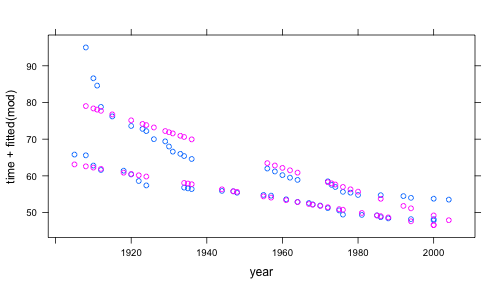With lm(), you can add in lots of variables and keep the confidence intervals small — there aren't so many “groups”. What's difficult is that the idea of “group” breaks down. So we'll have to learn to interpret the language of linear models.

## Form Groups for Car Project

Outline the used car project and show some data from http://www.cars.com. Perhaps MINI Coopers.

Get students to form groups right now, oriented around different kinds of cars. They should make a Google Spreadsheet and share it with all the other members of their group.

## The Overall Modeling Process

The modelling process, in this framework, is

1. Choose the response variable, based on the motivation you have for constructing your model.
2. Pick explanatory variables
3. Decide which terms to construct from the explanatory variables.
4. Fit the model — this is automatic, given (3) and the data.
5. Interpret the model, including consideration of the residuals. We'll spend a lot of time on this in the second half of the course.
6. Perhaps modify the model based on the information in (5) and go back to step (2). There may be many iterations of this.
7. Interpret the model in terms of the scientific or policy questions of interest. This may also involve iterating back to steps (1) or (2)

Right now, we are going to focus on step (3). For now, we'll pretend that (1) and (2) are obvious — but (2) especially can involve considerable nuance and debate. We'll also spend a bit of time on the techniques used in (7).

### The Math 135 Heuristic and two upcoming heuristics

Reminder of what “heuristic” means and why heuristics are useful.

Possible definition “Computing a solution by rules that are loosely defined.”

From Wikipedia:

Heuristic refers to experience-based techniques for problem solving, learning, and discovery. Where an exhaustive search is impractical, heuristic methods are used to speed up the process of finding a satisfactory solution. Examples of this method include using a rule of thumb, an educated guess, an intuitive judgment, or common sense.

In more precise terms, heuristics are strategies using readily accessible, though loosely applicable, information to control problem solving in human beings and machines.

We use heuristics to provide guidance and a ready way to move forward, while allowing room for judgment.

Back in Applied Calculus, we introduced a heuristic for including terms in a polynomial model.

• intercept and main effects: always
• quadratic terms (a particular type of transformation term): if we have a local, internal extremum.
• interaction term: if the effect of one input depends on the value of the other input.

That heuristic is still valid. In statistics, we'll be able to introduce another heuristic, based on the amount of evidence we have in the data to support the inclusion of any particular term. We won't be able to introduce this for a few weeks.

At the very end of the course, we'll introduce still another heuristic, based on the connections between variables in our theory of how the system we are modeling works.

## Introducing $$R^2$$ Briefly

Since we're trying to account for variation in the response variable, it's helpful to have a way to quantify how much variation we have accounted for. The quantity called “R-squared” — $$R^2$$, officially “the coefficient of determination” although hardly anyone uses that name — is a standard way to do this. Briefly, $$R^2$$ is the fraction of variance in the response variable that is “captured” by the model. It is always between 0 (didn't capture any) and 1 (got it all!).

### Activity

Visual choice of model terms

Instructions are given here (These have to be reformatted as a handout and as an instructor's guide.)

The only substantive differences is in the way the software is loaded:

fetchData("mLM.R")

## Retrieving from http://www.mosaic-web.org/go/datasets/mLM.R

##  TRUE


The program itself is called mLM() (not lm.select.terms())

## Model Formulas and Coefficients

Fit some models and work students through the calculations. The makeFun() function can help in this.

A suggested style for a calculation:

mod = lm(wage ~ sex + age, data = CPS85)
coef(mod)

## (Intercept)        sexM         age
##     4.65425     2.27469     0.08522


What is the wage for a female of age 40? A male of age 30? Work through the arithmetic “by hand”.

4.65 + 0 * 2.274 + 40 * 0.0852  # for the 40-year old female

##  8.058

4.65 + 1 * 2.274 + 30 * 0.0852  # for the 30-year old male

##  9.48


You can also turn the model information into a mathematical function:

f = makeFun(mod)
f(sex = "F", age = 40)

##     1
## 8.063

f(sex = "M", age = 30)

##     1
## 9.485


Other settings:

• Natural gas usage by average temperature in utilities.csv
• … more …